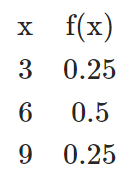# The following table provides a probability distribution for the random variable x

The following table provides a probability distribution for the random variable x.a. Compute E(x) , the expected value of x.
b. Compute σ2, the variance of x.
c. Compute σ, the standard deviation of x.